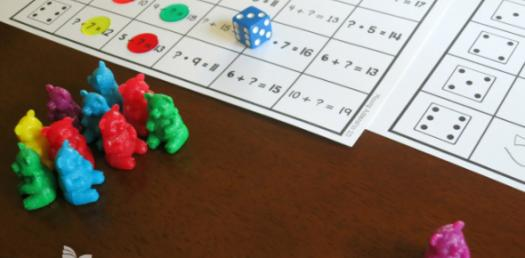# Solve This Addition Quiz If You Can! Test Your Math Skills

48 Questions | Total Attempts: 861SettingsSolve This Addition Quiz If You Can! If you are looking to test Your math skills, you are in luck as there are a number of quizzes dedicated to each function and the one below is on addition. These questions will give you the skills necessary to improve anyone’s math skills. Take our quiz and see how easy it is to solve them.

Questions and Answers
• 1.
3+2=
• 2.
2+1=
• 3.
5+0=
• 4.
4+1=
• 5.
2+3=
• 6.
1+0=
• 7.
0+4=
• 8.
3+2=
• 9.
2+2=
• 10.
2+3=
• 11.
3+0=
• 12.
1+2=
• 13.
5+0=
• 14.
4+1=
• 15.
2+1=
• 16.
3+0=
• 17.
3+2=
• 18.
4+1=
• 19.
2+0=
• 20.
0+5=
• 21.
4+0=
• 22.
3+2=
• 23.
2+2=
• 24.
1+3=
• 25.
1+4=
Related Topics